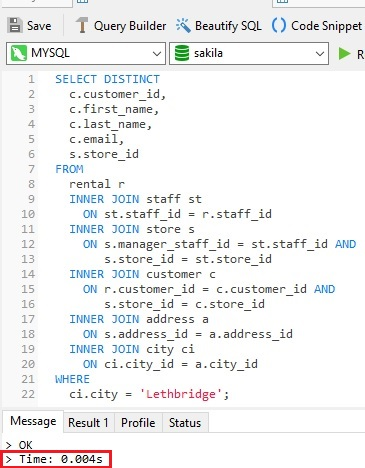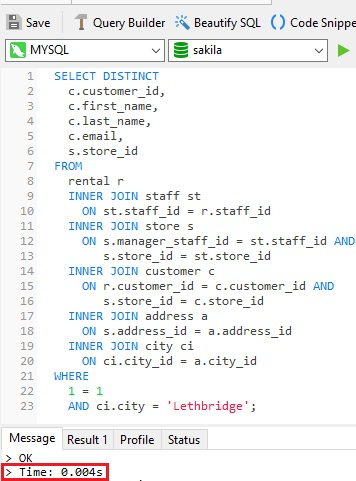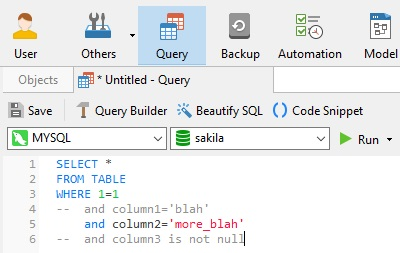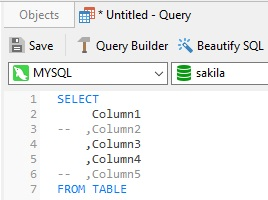Navicat Blog

The Purpose of WHERE 1=1 in SQL Statements Nov 8, 2021 by Robert Gravelle

Have you ever seen a WHERE 1=1 condition in a SELECT query. I have, within many different queries and across many SQL engines. The condition obviously means WHERE TRUE, so it's just returning the same query result as it would without the WHERE clause. Also, since the query optimizer would almost certainly remove it, there's no impact on query execution time. So, what is the purpose of the WHERE 1=1? That is the question that we're going to answer here today!

Does WHERE 1=1 Improve Query Execution?

As stated in the introduction, we would expect the query optimizer to remove the hard-coded WHERE 1=1 clause, so we should not see a reduced query execution time. To confirm this assumption, let's run a SELECT query in Navicat both with and without the WHERE 1=1 clause.

First, here's a query against the Sakila Sample Database that fetches customers who rented movies from the Lethbridge store:The execution time of 0.004 seconds (highlighted with a red outline) can bee seen at the bottom of the Messages tab.

Now, let's run the same query, except with the addition of the WHERE 1=1 clause:Again, the execution time was 0.004 seconds. Although a query's run time can fluctuate slightly, depending on many factors, it is safe to say that the WHERE 1=1 clause had no effect.

So, why use it then? Simply put, it's...

A Matter of Convenience

The truth of the matter is that the WHERE 1=1 clause is merely a convention adopted by some developers to make working with their SQL statements a little easier, both in static and dynamic form.

In Static SQL

When adding in conditions to a query that already has WHERE 1=1, all conditions thereafter will contain AND, so it's easier when commenting out conditions on experimental queries.This is similar to another technique where you'd have commas before column names rather than after. Again, it's easier for commenting:In Dynamic SQL

It's also a common practice when building an SQL query programmatically. It's easier to start with 'WHERE 1=1 ' and then append other criteria such as ' and customer.id=:custId', depending on whether or not a customer ID is provided. This allows the developer to append the next criterion in the query starting with 'and ...'. Here's a hypothetical example:

stmt  = "SELECT * "
stmt += "FROM TABLE "
stmt += "WHERE 1=1 "
if user chooses option a then stmt += "and A is not null "
if user chooses option b then stmt += "and B is not null "
if user chooses option b then stmt += "and C is not null "
if user chooses option b then stmt += "and D is not null "

Conclusion

In this blog, we learned the answer to the age-old question of "what is the purpose of the WHERE 1=1?" It's not an advanced optimization technique, but a style convention espoused by some developers.

Blog Archives
Share
• Key Topics• Products• Partners